Next: 4. AMIGOS Up: 3. Numerical Discretization Methods Previous: 3.1.3 Shape Functions

# 3.2 Finite Boxes

In contrast to the finite element discretization, which is a general mathematical motivated approach to solve complex differential equation mapped on a discretized simulation domain, the finite boxes are more physically motivated and especially in problems where a conservative law

 div() =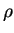(3.31)

is of importance the resulting approximations are of high quality [Fra83][Put98].

The idea is to calculate the flux across a defined volume using Gauss' integration method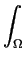div(F)dV =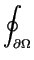=dV (3.32)

The mechanism of discretization in comparison to the finite element approach is rather simple because (3.32) can immediately be mapped to a numerical recipe and only geometrical calculations have to be made.

The integral (3.32) can be discretized with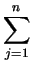Aij . Fij =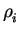. Vi (3.33)

where each node a box-volume is assigned to as depicted in Fig. 3.5.

The flux itself can be approximated by finite differences

 Fij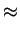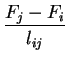(3.34)

such that the integral (3.32) for each inner point i finally results to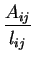. Fi =. Vi (3.35)

To calculate the sum in (3.35) the geometric coefficients Ai denoting the coupling cross section and lij which is the distance between the two corresponding discrete points [Fis94a] have to be found.The same procedure can be used calculating the discretization for three-dimensional problems where just the geometrical operations are more complicated than in the two-dimensional case [Mil94]. To meet the element-wise approach of finite elements the box can be calculated using the circumcenter M of the tetrahedron and the circumcenters of the triangular faces (Fig. 3.6). A Voronoi box in the three-dimensional case can be calculated by adding the resulting subtetrahedrons of all elements linked to a single point.

Due to the simple mapping mechanism between physics and discretization the finite box integration has been used very successfully in describing rather complex physical models such as semiconductor equations [Sim96] or coupled diffusion equations [Lei97].Next: 4. AMIGOS Up: 3. Numerical Discretization Methods Previous: 3.1.3 Shape Functions